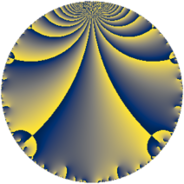# Properties

 Label 245.4.aLevel $245$ Weight $4$ Character orbit 245.a Rep. character $\chi_{245}(1,\cdot)$ Character field $\Q$ Dimension $41$ Newform subspaces $16$ Sturm bound $112$ Trace bound $3$

# Related objects

## Defining parameters

 Level: $$N$$ $$=$$ $$245 = 5 \cdot 7^{2}$$ Weight: $$k$$ $$=$$ $$4$$ Character orbit: $$[\chi]$$ $$=$$ 245.a (trivial) Character field: $$\Q$$ Newform subspaces: $$16$$ Sturm bound: $$112$$ Trace bound: $$3$$ Distinguishing $$T_p$$: $$2$$, $$3$$

## Dimensions

The following table gives the dimensions of various subspaces of $$M_{4}(\Gamma_0(245))$$.

Total New Old
Modular forms 92 41 51
Cusp forms 76 41 35
Eisenstein series 16 0 16

The following table gives the dimensions of the cuspidal new subspaces with specified eigenvalues for the Atkin-Lehner operators and the Fricke involution.

$$5$$$$7$$FrickeDim
$$+$$$$+$$$+$$$11$$
$$+$$$$-$$$-$$$9$$
$$-$$$$+$$$-$$$9$$
$$-$$$$-$$$+$$$12$$
Plus space$$+$$$$23$$
Minus space$$-$$$$18$$

## Trace form

 $$41 q + 2 q^{3} + 148 q^{4} + 5 q^{5} - 36 q^{8} + 341 q^{9} + O(q^{10})$$ $$41 q + 2 q^{3} + 148 q^{4} + 5 q^{5} - 36 q^{8} + 341 q^{9} + 40 q^{10} + 28 q^{11} + 188 q^{12} + 22 q^{13} - 50 q^{15} + 772 q^{16} + 170 q^{17} + 356 q^{18} - 344 q^{19} + 40 q^{20} - 32 q^{22} - 238 q^{23} - 44 q^{24} + 1025 q^{25} + 156 q^{26} - 76 q^{27} - 110 q^{29} - 240 q^{30} + 156 q^{31} - 1228 q^{32} - 360 q^{33} - 360 q^{34} + 1932 q^{36} - 1342 q^{37} + 500 q^{38} - 372 q^{39} + 240 q^{40} - 458 q^{41} - 694 q^{43} + 996 q^{44} - 275 q^{45} - 1148 q^{46} + 1058 q^{47} + 2028 q^{48} + 1308 q^{51} - 2072 q^{52} - 102 q^{53} - 788 q^{54} + 520 q^{55} + 2672 q^{57} - 2084 q^{58} + 1472 q^{59} + 260 q^{60} - 762 q^{61} + 552 q^{62} + 2540 q^{64} - 870 q^{65} - 1092 q^{66} + 2254 q^{67} - 2692 q^{68} - 204 q^{69} - 180 q^{71} + 1768 q^{72} - 190 q^{73} + 508 q^{74} + 50 q^{75} - 4084 q^{76} - 2136 q^{78} + 3880 q^{79} + 1120 q^{80} + 3733 q^{81} + 508 q^{82} + 2202 q^{83} + 30 q^{85} - 4408 q^{86} + 1684 q^{87} - 4784 q^{88} + 994 q^{89} + 2780 q^{90} - 6548 q^{92} - 2352 q^{93} + 444 q^{94} + 20 q^{95} + 6396 q^{96} - 4254 q^{97} - 7332 q^{99} + O(q^{100})$$

## Decomposition of $$S_{4}^{\mathrm{new}}(\Gamma_0(245))$$ into newform subspaces

Label Dim $A$ Field CM Traces A-L signs $q$-expansion
$a_{2}$ $a_{3}$ $a_{5}$ $a_{7}$ 5 7
245.4.a.a $1$ $14.455$ $$\Q$$ None $$-4$$ $$-2$$ $$5$$ $$0$$ $-$ $-$ $$q-4q^{2}-2q^{3}+8q^{4}+5q^{5}+8q^{6}+\cdots$$
245.4.a.b $1$ $14.455$ $$\Q$$ None $$1$$ $$-6$$ $$5$$ $$0$$ $-$ $-$ $$q+q^{2}-6q^{3}-7q^{4}+5q^{5}-6q^{6}+\cdots$$
245.4.a.c $1$ $14.455$ $$\Q$$ None $$1$$ $$6$$ $$-5$$ $$0$$ $+$ $-$ $$q+q^{2}+6q^{3}-7q^{4}-5q^{5}+6q^{6}+\cdots$$
245.4.a.d $1$ $14.455$ $$\Q$$ None $$1$$ $$8$$ $$5$$ $$0$$ $-$ $-$ $$q+q^{2}+8q^{3}-7q^{4}+5q^{5}+8q^{6}+\cdots$$
245.4.a.e $1$ $14.455$ $$\Q$$ None $$3$$ $$-2$$ $$5$$ $$0$$ $-$ $+$ $$q+3q^{2}-2q^{3}+q^{4}+5q^{5}-6q^{6}+\cdots$$
245.4.a.f $1$ $14.455$ $$\Q$$ None $$3$$ $$2$$ $$-5$$ $$0$$ $+$ $-$ $$q+3q^{2}+2q^{3}+q^{4}-5q^{5}+6q^{6}+\cdots$$
245.4.a.g $2$ $14.455$ $$\Q(\sqrt{2})$$ None $$-6$$ $$-2$$ $$10$$ $$0$$ $-$ $+$ $$q+(-3+\beta )q^{2}+(-1-3\beta )q^{3}+(3-6\beta )q^{4}+\cdots$$
245.4.a.h $2$ $14.455$ $$\Q(\sqrt{2})$$ None $$-6$$ $$2$$ $$-10$$ $$0$$ $+$ $-$ $$q+(-3+\beta )q^{2}+(1+3\beta )q^{3}+(3-6\beta )q^{4}+\cdots$$
245.4.a.i $2$ $14.455$ $$\Q(\sqrt{11})$$ None $$2$$ $$-10$$ $$-10$$ $$0$$ $+$ $-$ $$q+(1+\beta )q^{2}-5q^{3}+(4+2\beta )q^{4}-5q^{5}+\cdots$$
245.4.a.j $2$ $14.455$ $$\Q(\sqrt{11})$$ None $$2$$ $$10$$ $$10$$ $$0$$ $-$ $-$ $$q+(1+\beta )q^{2}+5q^{3}+(4+2\beta )q^{4}+5q^{5}+\cdots$$
245.4.a.k $2$ $14.455$ $$\Q(\sqrt{2})$$ None $$8$$ $$-2$$ $$10$$ $$0$$ $-$ $-$ $$q+(4+\beta )q^{2}+(-1+4\beta )q^{3}+(10+8\beta )q^{4}+\cdots$$
245.4.a.l $3$ $14.455$ 3.3.14360.1 None $$-3$$ $$-2$$ $$-15$$ $$0$$ $+$ $-$ $$q+(-1+\beta _{1})q^{2}+(-1-\beta _{1}+\beta _{2})q^{3}+\cdots$$
245.4.a.m $5$ $14.455$ $$\mathbb{Q}[x]/(x^{5} - \cdots)$$ None $$1$$ $$-8$$ $$-25$$ $$0$$ $+$ $+$ $$q+\beta _{1}q^{2}+(-2+\beta _{2})q^{3}+(7+\beta _{3})q^{4}+\cdots$$
245.4.a.n $5$ $14.455$ $$\mathbb{Q}[x]/(x^{5} - \cdots)$$ None $$1$$ $$8$$ $$25$$ $$0$$ $-$ $-$ $$q+\beta _{1}q^{2}+(2-\beta _{2})q^{3}+(7+\beta _{3})q^{4}+\cdots$$
245.4.a.o $6$ $14.455$ 6.6.1163891200.1 None $$-2$$ $$-16$$ $$30$$ $$0$$ $-$ $+$ $$q-\beta _{1}q^{2}+(-3+\beta _{5})q^{3}+(2+3\beta _{1}+\cdots)q^{4}+\cdots$$
245.4.a.p $6$ $14.455$ 6.6.1163891200.1 None $$-2$$ $$16$$ $$-30$$ $$0$$ $+$ $+$ $$q-\beta _{1}q^{2}+(3-\beta _{5})q^{3}+(2+3\beta _{1}+2\beta _{2}+\cdots)q^{4}+\cdots$$

## Decomposition of $$S_{4}^{\mathrm{old}}(\Gamma_0(245))$$ into lower level spaces

$$S_{4}^{\mathrm{old}}(\Gamma_0(245)) \cong$$ $$S_{4}^{\mathrm{new}}(\Gamma_0(5))$$$$^{\oplus 3}$$$$\oplus$$$$S_{4}^{\mathrm{new}}(\Gamma_0(7))$$$$^{\oplus 4}$$$$\oplus$$$$S_{4}^{\mathrm{new}}(\Gamma_0(35))$$$$^{\oplus 2}$$$$\oplus$$$$S_{4}^{\mathrm{new}}(\Gamma_0(49))$$$$^{\oplus 2}$$